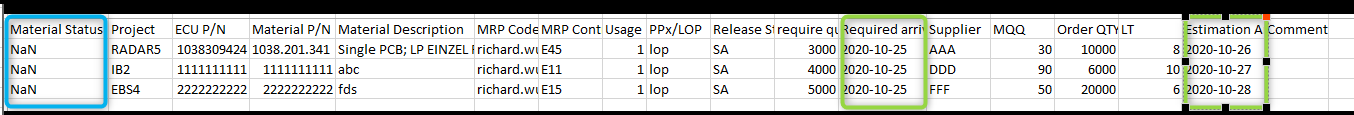# Apply formulas to columns get wrong result

Used apply formulas to columns to update one column "Material Status" within table, with columns['Material Status']=columns-columns, but the result shows NaN, shown as below• Hi @Richard_309702 ,
Thanks for reaching out with your question!

The NaN error your received stands for Not a Number, indicating the subtraction action couldn't be completed.

In this case you need to use `new Date()` to covert the dates into Date types and then find the difference.

Since the difference of two date types is in milliseconds, you will then need to divide by `(1000 * 60 * 60 * 24)` to get the difference in days. see below:

`result = new Date(columns['Date2']) - new Date(columns['Date1']);`

`result = result / (1000 * 60 * 60 * 24);`

`columns['Status']= result`